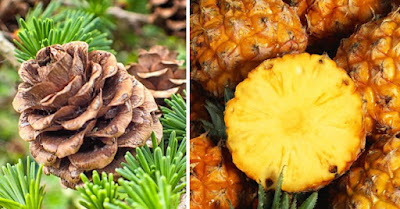## Tuesday, September 24, 2019

### Mother Nature's Favorite Number Sequence - The Fibonacci | Biology - Interesting Engineering

From pine cones to sunflowers, from nautilus shells to Dan Brown thrillers, mother nature has a favorite number sequence - the Fibonacci Sequence, explains Marcia Wendorf, former high school math teacher, technical writer, author, and programmer.Photo:
The spiral of seeds in a pine cone, the fruitlets of a pineapple. What do they have in common? They both conform to the Fibonacci Sequence.
As anyone who has read Dan Brown's thriller The Da Vinci Code or seen the movie knows, the Fibonacci Sequence is a sequence of numbers created by adding two sequential integers together, starting at 0.

The sequence can be described by the equation: Fn = Fn - 1 + Fn - 2, where n > 1 so, F0 = 0, F1 = 1 and F2 = F1 + F0 = 1.
The sequence of numbers comprising the Fibonacci Sequence is: 0, 1, 1, 2, 3, 5, 8, 13, 21, 34, 55, 89, 144, 233, 377, 610, 987 ...

Nicknamed Fibonacci
The person who brought the Fibonacci Sequence to Western audiences is Leonardo of Pisa, who was born around 1170 A.D. and died around 1250 A.D. He was later nicknamed Fibonacci, from Filius Bonacci, which means 'son of Bonacci'. The sequence had actually been deduced by Indian and Arab mathematicians a thousand years earlier.

In 1202, Fibonacci described the sequence in his Liber Abaci ('Book of Calculation'), which was intended as a math guide for tradesmen, so they could calculate profit and loss, and loan balances...

Finally, have you ever noticed that the covers of many high school mathematics textbooks display a nautilus shell? The shell can be described as having a spiral that expands by the Golden Ratio every 180 degrees. Although this is just an approximation, it is often cited as a sign of the appearance of the Golden Ratio in nature, and that's why it's on the cover of math textbooks.

Source: Interesting Engineering